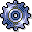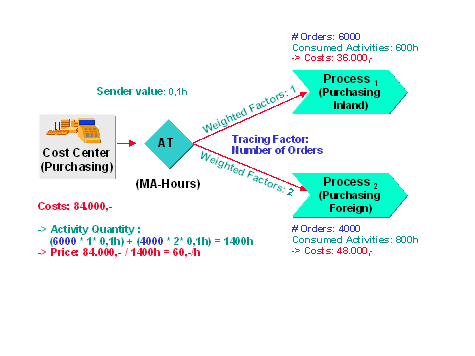Indirect Activity AllocationUse

Indirect activity allocation automatically assigns activity quantities in plan and actual. Unlike manual planning or actual activity allocation, you define keys to allocate the activities. In addition, if calculating the sender activity quantities involves too much time or expense, the R/3 System can determine this value inversely based on the receiver activity quantities.

Target=actual allocation is a special form of indirect activity allocation in actual. In contrast with the other forms of indirect activity allocation, target=actual allocation allows you to create an iterative activity input network with multiple levels using the operating rate as the tracing factor.

Features

You may use two approaches in indirect activity allocation, depending on the activity category. You define these approaches using segments, which you can combine in cycles.

• Posting activity quantities on the sender object allowed

Certain activity types allow you to determine the total activity provided per sender. These activity types belong to category 3 (manual entry, indirect allocation). Indirect activity allocation assigns the activity quantity from the sender to the receivers defined in the segment according to the specified tracing factor.

The segment must use the sender rule Posted quantities. Any receiver rule can be used with the exception of Fixed quantities.

• Posting activity quantities is difficult or impossible

For those activity types where it is impractical to calculate activity quantities, the R/3 System determines the activity quantity based on:

• Receiver tracing factors with weightings defined for each sender
• Segment definition (Fixed quantities)

These activity types belong to category 2 (indirect entry, indirect allocation).

The segment must use the sender rule Quantities determined indirectly with any receiver rule or the sender/receiver rule Fixed quantities.

If you use Quantities determined indirectly, the weightings described above are defined using Sender values.

Example

The numbers of orders executed by receiver processes (inland purchases) 1 and 2 (foreign purchases) are given in the illustration; inland 6000 orders, foreign 4000 orders.

Calculation of the consumed activity quantities: because the processing of the foreign orders is expensive, the system assigns it a weighted factor of 2. The activity quantity is calculated from sender value 0,1 h (base processing time per order), weighted factor and consumed activity (bottom, left part of the illustration).

Cost calculations: the price per hour is the result of dividing the total costs by the activity quantity. This value is then multiplied with the consumes activity.

(600 * 60.- = 36,000.- , or 800 * 60.- = 48,000.-).